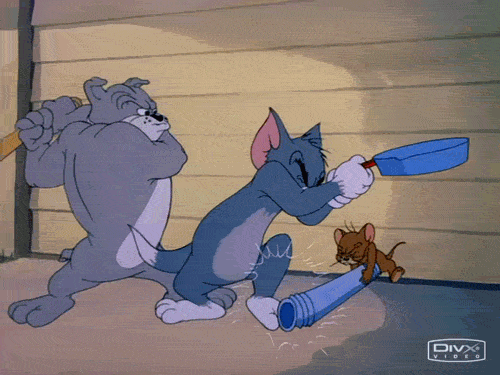# SAT Math V

Algebra Level 2If $x^2-x=12$ and $y^2-y=12$, what is the greatest possible value of $x-y$?

×